x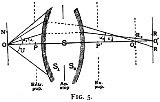Aberration in optical systemsEncyclopedia
Aberrations are departures of the performance of an optical system from the predictions of paraxial optics. Aberration leads to blurring of the image produced by an image-forming optical system. It occurs when light from one point of an object after transmission through the system does not converge into (or does not diverge from) a single point. Instrument-makers need to correct optical systems to compensate for aberration. The articles on reflection
Reflection (physics)
Reflection is the change in direction of a wavefront at an interface between two differentmedia so that the wavefront returns into the medium from which it originated. Common examples include the reflection of light, sound and water waves...

, refraction
Refraction
Refraction is the change in direction of a wave due to a change in its speed. It is essentially a surface phenomenon . The phenomenon is mainly in governance to the law of conservation of energy. The proper explanation would be that due to change of medium, the phase velocity of the wave is changed...

and caustics
Caustic (optics)
In optics, a caustic or caustic network is the envelope of light rays reflected or refracted by a curved surface or object, or the projection of that envelope of rays on another surface. The caustic is a curve or surface to which each of the light rays is tangent, defining a boundary of an...

discuss the general features of reflected and refracted rays
Ray (optics)
In optics, a ray is an idealized narrow beam of light. Rays are used to model the propagation of light through an optical system, by dividing the real light field up into discrete rays that can be computationally propagated through the system by the techniques of ray tracing. This allows even very...

.

## Overview

Aberrations fall into two classes: monochromatic and chromatic. Monochromatic aberrations are caused by the geometry of the lens and occur both when light is reflected and when it is refracted. They appear even when using monochromatic light, hence the name.

Chromatic aberration
Chromatic aberration
In optics, chromatic aberration is a type of distortion in which there is a failure of a lens to focus all colors to the same convergence point. It occurs because lenses have a different refractive index for different wavelengths of light...

s are caused by dispersion
Dispersion (optics)
In optics, dispersion is the phenomenon in which the phase velocity of a wave depends on its frequency, or alternatively when the group velocity depends on the frequency.Media having such a property are termed dispersive media...

, the variation of a lens's
Lens (optics)
A lens is an optical device with perfect or approximate axial symmetry which transmits and refracts light, converging or diverging the beam. A simple lens consists of a single optical element...

refractive index
Refractive index
In optics the refractive index or index of refraction of a substance or medium is a measure of the speed of light in that medium. It is expressed as a ratio of the speed of light in vacuum relative to that in the considered medium....

with wavelength
Wavelength
In physics, the wavelength of a sinusoidal wave is the spatial period of the wave—the distance over which the wave's shape repeats.It is usually determined by considering the distance between consecutive corresponding points of the same phase, such as crests, troughs, or zero crossings, and is a...

. They do not appear when monochromatic light is used.

### Monochromatic aberrations

• Piston
Piston (optics)
In optics, piston is the mean value of a wavefront or phase profile across the pupil of an optical system. The piston coefficient is typically expressed in wavelengths of light at a particular wavelength...

• Tilt
Tilt (optics)
In optics, tilt is a deviation in the direction a beam of light propagates. Tilt quantifies the average slope in both the X and Y directions of a wavefront or phase profile across the pupil of an optical system...

• Defocus
Defocus aberration
In optics, defocus is the aberration in which an image is simply out of focus. This aberration is familiar to anyone who has used a camera, videocamera, microscope, telescope, or binoculars. Optically, defocus refers to a translation along the optical axis away from the plane or surface of best...

• Spherical aberration
Spherical aberration
thumb|right|Spherical aberration. A perfect lens focuses all incoming rays to a point on the [[Optical axis|optic axis]]. A real lens with spherical surfaces suffers from spherical aberration: it focuses rays more tightly if they enter it far from the optic axis than if they enter closer to the...

• Coma
Coma (optics)
In optics , the coma in an optical system refers to aberration inherent to certain optical designs or due to imperfection in the lens or other components which results in off-axis point sources such as stars appearing distorted, appearing to have a tail like a comet...

• Astigmatism
Astigmatism
An optical system with astigmatism is one where rays that propagate in two perpendicular planes have different foci. If an optical system with astigmatism is used to form an image of a cross, the vertical and horizontal lines will be in sharp focus at two different distances...

• Field curvature
• Image distortion

Piston and tilt are not actually true optical aberrations, as they do not represent or model curvature
Curvature
In mathematics, curvature refers to any of a number of loosely related concepts in different areas of geometry. Intuitively, curvature is the amount by which a geometric object deviates from being flat, or straight in the case of a line, but this is defined in different ways depending on the context...

in the wavefront. If an otherwise perfect wavefront is "aberrated" by piston and tilt, it will still form a perfect, aberration-free image, only shifted to a different position. Defocus is the lowest-order true optical aberration.

### Chromatic aberrations

• Axial, or longitudinal, chromatic aberration
• Lateral, or transverse, chromatic aberration

## Monochromatic aberration

The elementary theory of optical systems leads to the theorem: Rays of light proceeding from any object point unite in an image point; and therefore an object space is reproduced in an image space. The introduction of simple auxiliary terms, due to C. F. Gauss
Carl Friedrich Gauss
Johann Carl Friedrich Gauss was a German mathematician and scientist who contributed significantly to many fields, including number theory, statistics, analysis, differential geometry, geodesy, geophysics, electrostatics, astronomy and optics.Sometimes referred to as the Princeps mathematicorum...

(Dioptrische Untersuchungen, Göttingen, 1841), named the focal length
Focal length
The focal length of an optical system is a measure of how strongly the system converges or diverges light. For an optical system in air, it is the distance over which initially collimated rays are brought to a focus...

s and focal planes, permits the determination of the image of any object for any system (see lens
Lens (optics)
A lens is an optical device with perfect or approximate axial symmetry which transmits and refracts light, converging or diverging the beam. A simple lens consists of a single optical element...

). The Gaussian theory, however, is only true so long as the angles made by all rays with the optical axis (the symmetrical axis of the system) are infinitely small, i.e. with infinitesimal objects, images and lenses; in practice these conditions are not realized, and the images projected by uncorrected systems are, in general, ill defined and often completely blurred, if the aperture or field of view exceeds certain limits.

The investigations of James Clerk Maxwell
James Clerk Maxwell
James Clerk Maxwell of Glenlair was a Scottish physicist and mathematician. His most prominent achievement was formulating classical electromagnetic theory. This united all previously unrelated observations, experiments and equations of electricity, magnetism and optics into a consistent theory...

(Phil.Mag., 1856; Quart. Journ. Math., 1858) and Ernst Abbe showed that the properties of these reproductions, i.e. the relative position and magnitude of the images, are not special properties of optical systems, but necessary consequences of the supposition (in Abbe) of the reproduction of all points of a space in image points (Maxwell assumes a less general hypothesis), and are independent of the manner in which the reproduction is effected. These authors proved, however, that no optical system can justify these suppositions, since they are contradictory to the fundamental laws of reflexion and refraction. Consequently the Gaussian theory only supplies a convenient method of approximating to reality; and no constructor would attempt to realize this unattainable ideal. All that at present can be attempted is, to reproduce a single plane in another plane; but even this has not been altogether satisfactorily accomplished, aberrations always occur, and it is improbable that these will ever be entirely corrected.

This, and related general questions, have been treated — besides the above-mentioned authors — by M. Thiesen (Berlin. Akad. Sitzber., 1890, xxxv. 799; Berlin. Phys. Ges. Verh., 1892) and H. Bruns (Leipzig. Math. Phys. Ber., 1895, xxi. 325) by means of Sir W. R. Hamilton's characteristic function (Irish Acad. Trans., Theory of Systems of Rays, 1828, et seq.). Reference may also be made to the treatise of Czapski-Eppenstein, pp. 155–161.

A review of the simplest cases of aberration will now be given.

### Aberration of axial points (spherical aberration in the restricted sense)

Let S (fig.5) be any optical system, rays proceeding from an axis point O under an angle u1 will unite in the axis point O'1; and those under an angle u2 in the axis point O'2. If there is refraction at a collective spherical surface, or through a thin positive lens, O'2 will lie in front of O'1 so long as the angle u2 is greater than u1 (under correction); and conversely with a dispersive surface or lenses (over correction). The caustic, in the first case, resembles the sign > (greater than); in the second < (less than). If the angle u1 is very small, O'1 is the Gaussian image; and O'1 O'2 is termed the longitudinal aberration, and O'1R the lateral aberration of the pencils with aperture u2. If the pencil with the angle u2 is that of the maximum aberration of all the pencils transmitted, then in a plane perpendicular to the axis at O'1 there is a circular disk of confusion of radius O'1R, and in a parallel plane at O'2 another one of radius O'2R2; between these two is situated the disk of least confusion.

The largest opening of the pencils, which take part in the reproduction of O, i.e. the angle u, is generally determined by the margin of one of the lenses or by a hole in a thin plate placed between, before, or behind the lenses of the system. This hole is termed the stop or diaphragm; Abbe used the term aperture
Aperture
In optics, an aperture is a hole or an opening through which light travels. More specifically, the aperture of an optical system is the opening that determines the cone angle of a bundle of rays that come to a focus in the image plane. The aperture determines how collimated the admitted rays are,...

stop
for both the hole and the limiting margin of the lens. The component S1 of the system, situated between the aperture stop and the object O, projects an image of the diaphragm, termed by Abbe the entrance pupil; the exit pupil is the image formed by the component S2, which is placed behind the aperture stop. All rays which issue from O and pass through the aperture stop also pass through the entrance and exit pupils, since these are images of the aperture stop. Since the maximum aperture of the pencils issuing from O is the angle u subtended by the entrance pupil at this point, the magnitude of the aberration will be determined by the position and diameter of the entrance pupil. If the system be entirely behind the aperture stop, then this is itself the entrance pupil (front stop); if entirely in front, it is the exit pupil (back stop).

If the object point be infinitely distant, all rays received by the first member of the system are parallel, and their intersections, after traversing the system, vary according to their perpendicular height of incidence, i.e. their distance from the axis. This distance replaces the angle u in the preceding considerations; and the aperture, i.e. the radius of the entrance pupil, is its maximum value.

### Aberration of elements, i.e. smallest objects at right angles to the axis

If rays issuing from O (fig. 5) be concurrent, it does not follow that points in a portion of a plane perpendicular at O to the axis will be also concurrent, even if the part of the plane be very small. With a considerable aperture, the neighboring point N will be reproduced, but attended by aberrations comparable in magnitude to ON. These aberrations are avoided if, according to Abbe, the sine condition, sin u'1/sin u1=sin u'2/sin u2, holds for all rays reproducing the point O. If the object point O is infinitely distant, u1 and u2 are to be replaced by h1 and h2, the perpendicular heights of incidence; the sine condition then becomes sin u'1/h1 sin u'2/h2. A system fulfilling this condition and free from spherical aberration is called aplanatic (Greek a-, privative, plann, a wandering). This word was first used by Robert Blair
Robert Blair (astronomer)
Robert Blair was a Scottish astronomer.Robert Blair was the first Regius Professor of Astronomy at the University of Edinburgh . He invented the aplanatic lens and also coined this term. This was a significant step in reducing the aberration in optical systems...

(d. 1828), professor of practical astronomy at Edinburgh University, to characterize a superior achromatism, and, subsequently, by many writers to denote freedom from spherical aberration. Both the aberration of axis points, and the deviation from the sine condition, rapidly increase in most (uncorrected) systems with the aperture.

### Aberration of lateral object points (points beyond the axis) with narrow pencils. Astigmatism.A point O (fig. 6) at a finite distance from the axis (or with an infinitely distant object, a point which subtends a finite angle at the system) is, in general, even then not sharply reproduced, if the pencil of rays issuing from it and traversing the system is made infinitely narrow by reducing the aperture stop; such a pencil consists of the rays which can pass from the object point through the now infinitely small entrance pupil. It is seen (ignoring exceptional cases) that the pencil does not meet the refracting or reflecting surface at right angles; therefore it is astigmatic (Gr. a-, privative, stigmia, a point). Naming the central ray passing through the entrance pupil the axis of the pencil or principal ray, it can be said: the rays of the pencil intersect, not in one point, but in two focal lines, which can be assumed to be at right angles to the principal ray; of these, one lies in the plane containing the principal ray and the axis of the system, i.e. in the first principal section or meridional section, and the other at right angles to it, i.e. in the second principal section or sagittal section. We receive, therefore, in no single intercepting plane behind the system, as, for example, a focusing screen, an image of the object point; on the other hand, in each of two planes lines O' and O" are separately formed (in neighboring planes ellipses are formed), and in a plane between O' and O" a circle of least confusion. The interval O'O", termed the astigmatic difference, increases, in general, with the angle W made by the principal ray OP with the axis of the system, i.e. with the field of view. Two astigmatic image surfaces correspond to one object plane; and these are in contact at the axis point; on the one lie the focal lines of the first kind, on the other those of the second. Systems in which the two astigmatic surfaces coincide are termed anastigmatic or stigmatic.

Sir Isaac Newton
Isaac Newton
Sir Isaac Newton PRS was an English physicist, mathematician, astronomer, natural philosopher, alchemist, and theologian, who has been "considered by many to be the greatest and most influential scientist who ever lived."...

was probably the discoverer of astigmation; the position of the astigmatic image lines was determined by Thomas Young (A Course of Lectures on Natural Philosophy, 1807); and the theory was developed by Allvar Gullstrand
Allvar Gullstrand
Allvar Gullstrand was a Swedish ophthalmologist.Born at Landskrona, Sweden, Gullstrand was professor successively of eye therapy and of optics at the University of Uppsala. He applied the methods of physical mathematics to the study of optical images and of the refraction of light in the eye...

. A bibliography by P. Culmann is given in Moritz von Rohr's Die Bilderzeugung in optischen Instrumenten.

### Aberration of lateral object points with broad pencils. Coma.

By opening the stop wider, similar deviations arise for lateral points as have been already discussed for axial points; but in this case they are much more complicated. The course of the rays in the meridional section is no longer symmetrical to the principal ray of the pencil; and on an intercepting plane there appears, instead of a luminous point, a patch of light, not symmetrical about a point, and often exhibiting a resemblance to a comet having its tail directed towards or away from the axis. From this appearance it takes its name. The unsymmetrical form of the meridional pencil—formerly the only one considered—is coma
Coma (optics)
In optics , the coma in an optical system refers to aberration inherent to certain optical designs or due to imperfection in the lens or other components which results in off-axis point sources such as stars appearing distorted, appearing to have a tail like a comet...

in the narrower sense only; other errors of coma have been treated by Arthur König
Arthur König
Arthur Peter König devoted his short life to physiological optics. Born with congenital kyphosis he studied in Bonn and Heidelberg, moving to Berlin in the fall of 1879 where he studied under Hermann von Helmholtz, whose assistant he became in 1882...

and Moritz von Rohr, and later by Allvar Gullstrand.

### Curvature of the field of the image

If the above errors be eliminated, the two astigmatic surfaces united, and a sharp image obtained with a wide aperture—there remains the necessity to correct the curvature of the image surface, especially when the image is to be received upon a plane surface, e.g. in photography. In most cases the surface is concave towards the system.

### Distortion of the imageEven if the image is sharp, it may be distorted compared to ideal pinhole projection. In pinhole projection, the magnification of an object is inversely proportional to its distance to the camera along the optical axis so that a camera pointing directly at a flat surface reproduces that flat surface. Distortion can be thought of as stretching the image non-uniformly, or, equivalently, as a variation in magnification across the field. While "distortion" can include arbitrary deformation of an image, the most pronounced modes of distortion produced by conventional imaging optics is "barrel distortion", in which the center of the image is magnified more than the perimeter (figure 7a). The reverse, in which the perimeter is magnified more than the center, is known as "pincushion distortion" (figure 7b). This effect is called lens distortion or image distortion, and there are algorithms to correct it.

Systems free of distortion are called orthoscopic (orthos, right, skopein to look) or rectilinear (straight lines).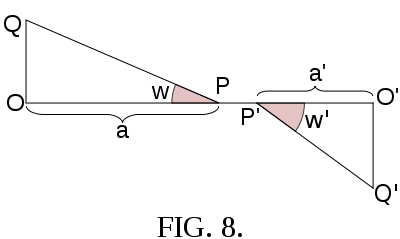This aberration is quite distinct from that of the sharpness of reproduction; in unsharp, reproduction, the question of distortion arises if only parts of the object can be recognized in the figure. If, in an unsharp image, a patch of light corresponds to an object point, the center of gravity of the patch may be regarded as the image point, this being the point where the plane receiving the image, e.g., a focusing screen, intersects the ray passing through the middle of the stop. This assumption is justified if a poor image on the focusing screen remains stationary when the aperture is diminished; in practice, this generally occurs. This ray, named by Abbe a principal ray (not to be confused with the principal rays of the Gaussian theory), passes through the center of the entrance pupil before the first refraction, and the center of the exit pupil after the last refraction. From this it follows that correctness of drawing depends solely upon the principal rays; and is independent of the sharpness or curvature of the image field. Referring to fig. 8, we have O'Q'/OQ = a' tan w'/a tan w = 1/N, where N is the scale or magnification of the image. For N to be constant for all values of w, a' tan w'/a tan w must also be constant. If the ratio a'/a be sufficiently constant, as is often the case, the above relation reduces to the condition of Airy
George Biddell Airy
Sir George Biddell Airy PRS KCB was an English mathematician and astronomer, Astronomer Royal from 1835 to 1881...

,
i.e. tan w'/ tan w= a constant. This simple relation (see Camb. Phil. Trans., 1830, 3, p. 1) is fulfilled in all systems which are symmetrical with respect to their diaphragm (briefly named symmetrical or holosymmetrical objectives), or which consist of two like, but different-sized, components, placed from the diaphragm in the ratio of their size, and presenting the same curvature to it (hemisymmetrical objectives); in these systems tan w' / tan w = 1.

The constancy of a'/a necessary for this relation to hold was pointed out by R. H. Bow (Brit. Journ. Photog., 1861), and Thomas Sutton (Photographic Notes, 1862); it has been treated by O. Lummer and by M. von Rohr (Zeit. f. Instrumentenk., 1897, 17, and 1898, 18, p. 4). It requires the middle of the aperture stop to be reproduced in the centers of the entrance and exit pupils without spherical aberration. M. von Rohr showed that for systems fulfilling neither the Airy nor the Bow-Sutton condition, the ratio a' cos w'/a tan w will be constant for one distance of the object. This combined condition is exactly fulfilled by holosymmetrical objectives reproducing with the scale 1, and by hemisymmetrical, if the scale of reproduction be equal to the ratio of the sizes of the two components.

### Zernike model of aberrations

Circular wavefront profiles associated with aberrations may be mathematically modeled using Zernike polynomials. Developed by Frits Zernike
Frits Zernike
Frits Zernike was a Dutch physicist and winner of the Nobel prize for physics in 1953 for his invention of the phase contrast microscope, an instrument that permits the study of internal cell structure without the need to stain and thus kill the cells....

in the 1930s, Zernike's polynomials are orthogonal over a circle of unit radius. A complex, aberrated wavefront profile may be curve-fitted with Zernike polynomials to yield a set of fitting coefficients that individually represent different types of aberrations. These Zernike coefficients are linearly independent, thus individual aberration contributions to an overall wavefront may be isolated and quantified separately.

There are even and odd
Even and odd functions
In mathematics, even functions and odd functions are functions which satisfy particular symmetry relations, with respect to taking additive inverses. They are important in many areas of mathematical analysis, especially the theory of power series and Fourier series...

Zernike polynomials. The even Zernike polynomials are defined as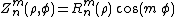and the odd Zernike polynomials as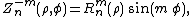where m and n are nonnegative integer
Integer
The integers are formed by the natural numbers together with the negatives of the non-zero natural numbers .They are known as Positive and Negative Integers respectively...

s with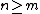,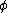is the azimuthal angle
Angle
In geometry, an angle is the figure formed by two rays sharing a common endpoint, called the vertex of the angle.Angles are usually presumed to be in a Euclidean plane with the circle taken for standard with regard to direction. In fact, an angle is frequently viewed as a measure of an circular arc...

Radian is the ratio between the length of an arc and its radius. The radian is the standard unit of angular measure, used in many areas of mathematics. The unit was formerly a SI supplementary unit, but this category was abolished in 1995 and the radian is now considered a SI derived unit...

s, and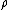is the normalized radial distance. The radial polynomials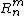have no azimuthal dependence, and are defined as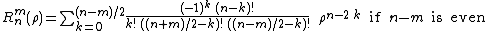and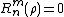ifis odd.

The first few Zernike polynomials are: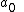"Piston", equal to the mean value of the wavefront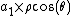"X-Tilt", the deviation of the overall beam in the sagittal direction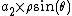"Y-Tilt", the deviation of the overall beam in the tangential direction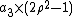"Defocus", a parabolicParabolaIn mathematics, the parabola is a conic section, the intersection of a right circular conical surface and a plane parallel to a generating straight line of that surface... wavefront resulting from being out of focus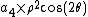"0° Astigmatism", a cylindrical shape along the X or Y axis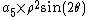"45° Astigmatism", a cylindrical shape oriented at ±45° from the X axis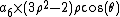"X-Coma", comatic image flaring in the horizontal direction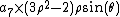"Y-Coma", comatic image flaring in the vertical direction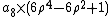"Third order spherical aberration"

whereis the normalized pupil radius with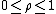,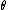is the azimuthal angle around the pupil with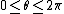, and the fitting coefficients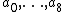are the wavefront errors in wavelengths.

As in Fourier synthesis using sine
Sine
In mathematics, the sine function is a function of an angle. In a right triangle, sine gives the ratio of the length of the side opposite to an angle to the length of the hypotenuse.Sine is usually listed first amongst the trigonometric functions....

s and cosines, a wavefront may be perfectly represented by a sufficiently large number of higher-order Zernike polynomials. However, wavefronts with very steep gradients or very high spatial frequency
Spatial frequency
In mathematics, physics, and engineering, spatial frequency is a characteristic of any structure that is periodic across position in space. The spatial frequency is a measure of how often sinusoidal components of the structure repeat per unit of distance. The SI unit of spatial frequency is...

structure, such as produced by propagation
Wave propagation
Wave propagation is any of the ways in which waves travel.With respect to the direction of the oscillation relative to the propagation direction, we can distinguish between longitudinal wave and transverse waves....

through atmospheric turbulence or aerodynamic flowfields
Turbulence
In fluid dynamics, turbulence or turbulent flow is a flow regime characterized by chaotic and stochastic property changes. This includes low momentum diffusion, high momentum convection, and rapid variation of pressure and velocity in space and time...

, are not well modeled by Zernike polynomials, which tend to low-pass filter
Low-pass filter
A low-pass filter is an electronic filter that passes low-frequency signals but attenuates signals with frequencies higher than the cutoff frequency. The actual amount of attenuation for each frequency varies from filter to filter. It is sometimes called a high-cut filter, or treble cut filter...

fine spatial definition in the wavefront. In this case, other fitting methods such as fractals or singular value decomposition
Singular value decomposition
In linear algebra, the singular value decomposition is a factorization of a real or complex matrix, with many useful applications in signal processing and statistics....

may yield improved fitting results.

The circle polynomials
Zernike polynomials
In mathematics, the Zernike polynomials are a sequence of polynomials that are orthogonal on the unit disk. Named after Frits Zernike, they play an important role in beam optics.-Definitions:There are even and odd Zernike polynomials...

were introduced by Fritz Zernike to evaluate the point image of an aberrated optical system taking into account the effects of diffraction
Diffraction
Diffraction refers to various phenomena which occur when a wave encounters an obstacle. Italian scientist Francesco Maria Grimaldi coined the word "diffraction" and was the first to record accurate observations of the phenomenon in 1665...

. The perfect point image in the presence of diffraction had already been described by Airy
George Biddell Airy
Sir George Biddell Airy PRS KCB was an English mathematician and astronomer, Astronomer Royal from 1835 to 1881...

, as early as 1835. It took almost hundred years to arrive at a comprehensive theory and modeling of the point image of aberrated systems (Zernike and Nijboer). The analysis by Nijboer and Zernike describes the intensity distribution close to the optimum focal plane. An extended theory that allows the calculation of the point image amplitude and intensity over a much larger volume in the focal region was recently developed (Extended Nijboer-Zernike theory). This Extended Nijboer-Zernike theory of point image or ‘point-spread function’ formation has found applications in general research on image formation, especially for systems with a high numerical aperture
Numerical aperture
In optics, the numerical aperture of an optical system is a dimensionless number that characterizes the range of angles over which the system can accept or emit light. By incorporating index of refraction in its definition, NA has the property that it is constant for a beam as it goes from one...

, and in characterizing optical systems with respect to their aberrations.

## Analytic treatment of aberrations

The preceding review of the several errors of reproduction belongs to the Abbe theory of aberrations, in which definite aberrations are discussed separately; it is well suited to practical needs, for in the construction of an optical instrument certain errors are sought to be eliminated, the selection of which is justified by experience. In the mathematical sense, however, this selection is arbitrary; the reproduction of a finite object with a finite aperture entails, in all probability, an infinite number of aberrations. This number is only finite if the object and aperture are assumed to be infinitely small of a certain order; and with each order of infinite smallness, i.e. with each degree of approximation to reality (to finite objects and apertures), a certain number of aberrations is associated. This connection is only supplied by theories which treat aberrations generally and analytically by means of indefinite series.
A ray proceeding from an object point O (fig. 9) can be defined by the coordinates (ξ, η). Of this point O in an object plane I, at right angles to the axis, and two other coordinates (x, y), the point in which the ray intersects the entrance pupil, i.e. the plane II. Similarly the corresponding image ray may be defined by the points (ξ', η'), and (x', y'), in the planes I' and II'. The origins of these four plane coordinate systems may be collinear with the axis of the optical system; and the corresponding axes may be parallel. Each of the four coordinates ξ', η', x', y' are functions of ξ, η, x, y; and if it be assumed that the field of view and the aperture be infinitely small, then ξ, η, x, y are of the same order of infinitesimals; consequently by expanding ξ', η', x', y' in ascending powers of ξ, η, x, y, series are obtained in which it is only necessary to consider the lowest powers. It is readily seen that if the optical system be symmetrical, the origins of the coordinate systems collinear with the optical axis and the corresponding axes parallel, then by changing the signs of ξ, η, x, y, the values ξ', η', x', y' must likewise change their sign, but retain their arithmetical values; this means that the series are restricted to odd powers of the unmarked variables.

The nature of the reproduction consists in the rays proceeding from a point O being united in another point O'; in general, this will not be the case, for ξ', η' vary if ξ, η be constant, but x, y variable. It may be assumed that the planes I' and II' are drawn where the images of the planes I and II are formed by rays near the axis by the ordinary Gaussian rules; and by an extension of these rules, not, however, corresponding to reality, the Gauss image point O'0, with coordinates ξ'0, η'0, of the point O at some distance from the axis could be constructed. Writing Dξ'=ξ'-ξ'0 and Dη'=η'-η'0, then Dξ' and Dη' are the aberrations belonging to ξ, η and x, y, and are functions of these magnitudes which, when expanded in series, contain only odd powers, for the same reasons as given above. On account of the aberrations of all rays which pass through O, a patch of light, depending in size on the lowest powers of ξ, η, x, y which the aberrations contain, will be formed in the plane I'. These degrees, named by (J. Petzval
Jozef Maximilián Petzval
Joseph Petzval was a Hungarian / Slovak mathematician, inventor, and physicist of German origin, born in Upper Hungary .He is best known for his work in optics...

(Bericht uber die Ergebnisse einiger dioptrischer Untersuchungen, Buda Pesth, 1843; Akad. Sitzber., Wien, 1857, vols. xxiv. xxvi.) the numerical orders of the image, are consequently only odd powers; the condition for the formation of an image of the mth order is that in the series for Dξ' and Dη' the coefficients of the powers of the 3rd, 5th…(m-2)th degrees must vanish. The images of the Gauss theory being of the third order, the next problem is to obtain an image of 5th order, or to make the coefficients of the powers of 3rd degree zero. This necessitates the satisfying of five equations; in other words, there are five alterations of the 3rd order, the vanishing of which produces an image of the 5th order.

The expression for these coefficients in terms of the constants of the optical system, i.e. the radii, thicknesses, refractive indices and distances between the lenses, was solved by L. Seidel
Philipp Ludwig von Seidel
Philipp Ludwig von Seidel was a German mathematician. His mother was Julie Reinhold and his father was Justus Christian Felix Seidel.Lakatos credits von Seidel with discovering, in 1847, the crucial analytic concept of uniform convergence, while analyzing an incorrect proof of Cauchy's.In 1857,...

(Astr. Nach., 1856, p. 289); in 1840, J. Petzval constructed his portrait objective, from similar calculations which have never been published (see M. von Rohr, Theorie und Geschichte des photographischen Objectivs, Berlin, 1899, p. 248). The theory was elaborated by S. Finterswalder (Munchen. Acad. Abhandl., 1891, 17, p. 519), who also published a posthumous paper of Seidel containing a short view of his work (München. Akad. Sitzber., 1898, 28, p. 395); a simpler form was given by A. Kerber (Beiträge zur Dioptrik, Leipzig, 1895-6-7-8-9). A. Konig and M. von Rohr (see M. von Rohr, Die Bilderzeugung in optischen Instrumenten, pp. 317–323) have represented Kerber's method, and have deduced the Seidel formulae from geometrical considerations based on the Abbe method, and have interpreted the analytical results geometrically (pp. 212–316).

The aberrations can also be expressed by means of the characteristic function of the system and its differential coefficients, instead of by the radii, &c., of the lenses; these formulae are not immediately applicable, but give, however, the relation between the number of aberrations and the order. Sir William Rowan Hamilton (British Assoc. Report, 1833, p. 360) thus derived the aberrations of the third order; and in later times the method was pursued by Clerk Maxwell (Proc. London Math. Soc., 1874–1875; (see also the treatises of R. S. Heath and L. A. Herman), M. Thiesen (Berlin. Akad. Sitzber., 1890, 35, p. 804), H. Bruns (Leipzig. Math. Phys. Ber., 1895, 21, p. 410), and particularly successfully by K. Schwarzschild (Göttingen. Akad. Abhandl., 1905, 4, No. 1), who thus discovered the aberrations of the 5th order (of which there are nine), and possibly the shortest proof of the practical (Seidel) formulae. A. Gullstrand (vide supra, and Ann. d. Phys., 1905, 18, p. 941) founded his theory of aberrations on the differential geometry of surfaces.

The aberrations of the third order are: (1) aberration of the axis point; (2) aberration of points whose distance from the axis is very small, less than of the third order — the deviation from the sine condition and coma here fall together in one class; (3) astigmatism; (4) curvature of the field; (5) distortion.
(1) Aberration of the third order of axis points is dealt with in all text-books on optics. It is very important in telescope design. In telescopes aperture is usually taken as the linear diameter of the objective. It is not the same as microscope aperture which is based on the entrance pupil or field of view as seen from the object and is expressed as an angular measurement. Higher order aberrations in telescope design can be mostly neglected. For microscopes it cannot be neglected. For a single lens of very small thickness and given power, the aberration depends upon the ratio of the radii r:r', and is a minimum (but never zero) for a certain value of this ratio; it varies inversely with the refractive index (the power of the lens remaining constant). The total aberration of two or more very thin lenses in contact, being the sum of the individual aberrations, can be zero. This is also possible if the lenses have the same algebraic sign. Of thin positive lenses with n=1.5, four are necessary to correct spherical aberration of the third order. These systems, however, are not of great practical importance. In most cases, two thin lenses are combined, one of which has just so strong a positive aberration (under-correction, vide supra) as the other a negative; the first must be a positive lens and the second a negative lens; the powers, however: may differ, so that the desired effect of the lens is maintained. It is generally an advantage to secure a great refractive effect by several weaker than by one high-power lens. By one, and likewise by several, and even by an infinite number of thin lenses in contact, no more than two axis points can be reproduced without aberration of the third order. Freedom from aberration for two axis points, one of which is infinitely distant, is known as Herschel's condition. All these rules are valid, inasmuch as the thicknesses and distances of the lenses are not to be taken into account.

(2) The condition for freedom from coma in the third order is also of importance for telescope objectives; it is known as Fraunhofer's
Joseph von Fraunhofer
Joseph von Fraunhofer was a German optician. He is known for the discovery of the dark absorption lines known as Fraunhofer lines in the Sun's spectrum, and for making excellent optical glass and achromatic telescope objectives.-Biography:Fraunhofer was born in Straubing, Bavaria...

condition.
(4) After eliminating the aberration On the axis, coma and astigmatism, the relation for the flatness of the field in the third order is expressed by the Petzval equation, S1/r(n'-n) = 0, where r is the radius of a refracting surface, n and n' the refractive indices of the neighboring media, and S the sign of summation for all refracting surfaces.

## Practical elimination of aberrations

The classical imaging problem is to reproduce perfectly a finite plane (the object) onto another plane (the image) through a finite aperture. It is impossible to do so perfectly for more than one such pairs of planes (this was proven with increasing generality by Maxwell
James Clerk Maxwell
James Clerk Maxwell of Glenlair was a Scottish physicist and mathematician. His most prominent achievement was formulating classical electromagnetic theory. This united all previously unrelated observations, experiments and equations of electricity, magnetism and optics into a consistent theory...

in 1858, by Bruns in 1895, and by Carathéodory
Constantin Carathéodory
Constantin Carathéodory was a Greek mathematician. He made significant contributions to the theory of functions of a real variable, the calculus of variations, and measure theory...

in 1926, see summary in Walther, A., J. Opt. Soc. Am. A 6, 415–422 (1989)). For a single pair of planes (e.g. for a single focus setting of an objective), however, the problem can in principle be solved perfectly. Examples of such a theoretically perfect system include the Luneburg lens and the Maxwell fish-eye.

Practical methods solve this problem with an accuracy which mostly suffices for the special purpose of each species of instrument. The problem of finding a system which reproduces a given object upon a given plane with given magnification (insofar as aberrations must be taken into account) could be dealt with by means of the approximation theory; in most cases, however, the analytical difficulties were too great for older calculation methods but may be ameliorated by application of modern computer systems. Solutions, however, have been obtained in special cases (see A. Konig in M. von Rohr's Die Bilderzeugung, p. 373; K. Schwarzschild, Göttingen. Akad. Abhandl., 1905, 4, Nos. 2 and 3). At the present time constructors almost always employ the inverse method: they compose a system from certain, often quite personal experiences, and test, by the trigonometrical calculation of the paths of several rays, whether the system gives the desired reproduction (examples are given in A. Gleichen, Lehrbuch der geometrischen Optik, Leipzig and Berlin, 1902). The radii, thicknesses and distances are continually altered until the errors of the image become sufficiently small. By this method only certain errors of reproduction are investigated, especially individual members, or all, of those named above. The analytical approximation theory is often employed provisionally, since its accuracy does not generally suffice.

In order to render spherical aberration and the deviation from the sine condition small throughout the whole aperture, there is given to a ray with a finite angle of aperture u* (width infinitely distant objects: with a finite height of incidence h*) the same distance of intersection, and the same sine ratio as to one neighboring the axis (u* or h* may not be much smaller than the largest aperture U or H to be used in the system). The rays with an angle of aperture smaller than u* would not have the same distance of intersection and the same sine ratio; these deviations are called zones, and the constructor endeavors to reduce these to a minimum. The same holds for the errors depending upon the angle of the field of view, w: astigmatism, curvature of field and distortion are eliminated for a definite value, w*, zones of astigmatism, curvature of field and distortion, attend smaller values of w. The practical optician names such systems: corrected for the angle of aperture u* (the height of incidence h*) or the angle of field of view w*. Spherical aberration and changes of the sine ratios are often represented graphically as functions of the aperture, in the same way as the deviations of two astigmatic image surfaces of the image plane of the axis point are represented as functions of the angles of the field of view.

The final form of a practical system consequently rests on compromise; enlargement of the aperture results in a diminution of the available field of view, and vice versa. But the larger aperture will give the larger resolution. The following may be regarded as typical:
Largest aperture; necessary corrections are — for the axis point, and sine condition; errors of the field of view are almost disregarded; example — high-power microscope objectives.
Wide angle lens; necessary corrections are — for astigmatism, curvature of field and distortion; errors of the aperture only slightly regarded; examples — photographic widest angle objectives and oculars.
Between these extreme examples stands the normal lens
Normal lens
In photography and cinematography a normal lens, also called a standard lens, is a lens that reproduces perspective that generally looks "natural" to a human observer under normal viewing conditions, as compared with lenses with longer or shorter focal lengths which produce an expanded or...

: this is corrected more with regard to aperture; objectives for groups more with regard to the field of view.

Long focus lenses have small fields of view and aberrations on axis are very important. Therefore zones will be kept as small as possible and design should emphasize simplicity. Because of this these lenses are the best for analytical computation.

## Chromatic or color aberration

In optical systems composed of lenses, the position, magnitude and errors of the image depend upon the refractive indices of the glass employed (see Lens (optics)
Lens (optics)
A lens is an optical device with perfect or approximate axial symmetry which transmits and refracts light, converging or diverging the beam. A simple lens consists of a single optical element...

and Monochromatic aberration, above). Since the index of refraction varies with the color or wavelength of the light (see dispersion
Dispersion (optics)
In optics, dispersion is the phenomenon in which the phase velocity of a wave depends on its frequency, or alternatively when the group velocity depends on the frequency.Media having such a property are termed dispersive media...

), it follows that a system of lenses (uncorrected) projects images of different colors in somewhat different places and sizes and with different aberrations; i.e. there are chromatic differences of the distances of intersection, of magnifications, and of monochromatic aberrations. If mixed light be employed (e.g. white light) all these images are formed; and since they are all ultimately intercepted by a plane (the retina of the eye, a focusing screen of a camera, etc.), they cause a confusion, named chromatic aberration; for instance, instead of a white margin on a dark background, there is perceived a colored margin, or narrow spectrum. The absence of this error is termed achromatism, and an optical system so corrected is termed achromatic. A system is said to be chromatically under-corrected when it shows the same kind of chromatic error as a thin positive lens, otherwise it is said to be over-corrected.

If, in the first place, monochromatic aberrations be neglected — in other words, the Gaussian theory be accepted — then every reproduction is determined by the positions of the focal planes, and the magnitude of the focal lengths, or if the focal lengths, as ordinarily happens, be equal, by three constants of reproduction. These constants are determined by the data of the system (radii, thicknesses, distances, indices, etc., of the lenses); therefore their dependence on the refractive index, and consequently on the color, are calculable. The refractive indices for different wavelengths must be known for each kind of glass made use of. In this manner the conditions are maintained that any one constant of reproduction is equal for two different colors, i.e. this constant is achromatized. For example, it is possible, with one thick lens in air, to achromatize the position of a focal plane of the magnitude of the focal length. If all three constants of reproduction be achromatized, then the Gaussian image for all distances of objects is the same for the two colors, and the system is said to be in stable achromatism.

In practice it is more advantageous (after Abbe) to determine the chromatic aberration (for instance, that of the distance of intersection) for a fixed position of the object, and express it by a sum in which each component conlins the amount due to each refracting surface. In a plane containing the image point of one color, another colour produces a disk of confusion; this is similar to the confusion caused by two zones in spherical aberration. For infinitely distant objects the radius Of the chromatic disk of confusion is proportional to the linear aperture, and independent of the focal length (vide supra, Monochromatic Aberration of the Axis Point); and since this disk becomes the less harmful with an increasing image of a given object, or with increasing focal length, it follows that the deterioration of the image is proportional to the ratio of the aperture to the focal length, i.e. the relative aperture. (This explains the gigantic focal lengths in vogue before the discovery of achromatism.)

Examples:
(a) In a very thin lens, in air, only one constant of reproduction is to be observed, since the focal length and the distance of the focal point are equal. If the refractive index for one color be, and for another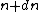, and the powers, or reciprocals of the focal lengths, be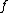and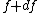, then (1)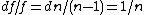;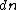is called the dispersion, andthe dispersive power of the glass.

(b) Two thin lenses in contact: let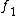and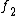be the powers corresponding to the lenses of refractive indices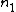and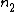and radii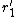,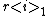, and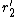,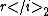respectively; letdenote the total power, and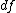,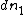,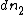the changes of,, andwith the color. Then the following relations hold:

(2)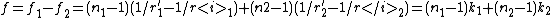; and

(3)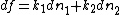. For achromatism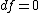, hence, from (3),

(4)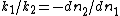, or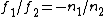. Thereforeandmust have different algebraic signs, or the system must be composed of a collective and a dispersive lens. Consequently the powers of the two must be different (in order thatbe not zero (equation 2)), and the dispersive powers must also be different (according to 4).

Newton failed to perceive the existence of media of different dispersive powers required by achromatism; consequently he constructed large reflectors instead of refractors. James Gregory and Leonhard Euler arrived at the correct view from a false conception of the achromatism of the eye; this was determined by Chester More Hall in 1728, Klingenstierna in 1754 and by Dollond in 1757, who constructed the celebrated achromatic telescopes. (See telescope
Telescope
A telescope is an instrument that aids in the observation of remote objects by collecting electromagnetic radiation . The first known practical telescopes were invented in the Netherlands at the beginning of the 1600s , using glass lenses...

.)

Glass with weaker dispersive power (greater) is named crown glass
Crown glass (optics)
Crown glass is type of optical glass used in lenses and other optical components. It has relatively low refractive index and low dispersion...

; that with greater dispersive power, flint glass
Flint glass
Flint glass is optical glass that has relatively high refractive index and low Abbe number. Flint glasses are arbitrarily defined as having an Abbe number of 50 to 55 or less. The currently known flint glasses have refractive indices ranging between 1.45 and 2.00...

. For the construction of an achromatic collective lens (positive) it follows, by means of equation (4), that a collective lens I. of crown glass and a dispersive lens II. of flint glass must be chosen; the latter, although the weaker, corrects the other chromatically by its greater dispersive power. For an achromatic dispersive lens the converse must be adopted. This is, at the present day, the ordinary type, e.g., of telescope objective (fig. 10); the values of the four radii must satisfy the equations (2) and (4). Two other conditions may also be postulated: one is always the elimination of the aberration on the axis; the second either the Herschel or Fraunhofer Condition, the latter being the best vide supra, Monochromatic Aberration). In practice, however, it is often more useful to avoid the second condition by making the lenses have contact, i.e. equal radii. According to P. Rudolph (Eder's Jahrb. f. Photog., 1891, 5, p. 225; 1893, 7, p. 221), cemented objectives of thin lenses permit the elimination of spherical aberration on the axis, if, as above, the collective lens has a smaller refractive index; on the other hand, they permit the elimination of astigmatism and curvature of the field, if the collective lens has a greater refractive index (this follows from the Petzval equation; see L. Seidel, Astr. Nachr., 1856, p. 289). Should the cemented system be positive, then the more powerful lens must be positive; and, according to (4), to the greater power belongs the weaker dispersive power (greater), that is to say, crown glass; consequently the crown glass must have the greater refractive index for astigmatic and plane images. In all earlier kinds of glass, however, the dispersive power increased with the refractive index; that is,decreased asincreased; but some of the Jena glasses by E. Abbe and O. Schott were crown glasses of high refractive index, and achromatic systems from such crown glasses, with flint glasses of lower refractive index, are called the new achromats, and were employed by P. Rudolph in the first anastigmats (photographic objectives).

Instead of makingvanish, a certain value can be assigned to it which will produce, by the addition of the two lenses, any desired chromatic deviation, e.g. sufficient to eliminate one present in other parts of the system. If the lenses I. and II. be cemented and have the same refractive index for one color, then its effect for that one color is that of a lens of one piece; by such decomposition of a lens it can be made chromatic or achromatic at will, without altering its spherical effect. If its chromatic effect (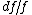) be greater than that of the same lens, this being made of the more dispersive of the two glasses employed, it is termed hyper-chromatic.

For two thin lenses separated by a distance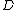the condition for achromatism is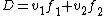; if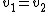(e.g. if the lenses be made of the same glass), this reduces to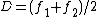, known as the condition for oculars.

If a constant of reproduction, for instance the focal length, be made equal for two colors, then it is not the same for other colors, if two different glasses are employed. For example, the condition for achromatism (4) for two thin lenses in contact is fulfilled in only one part of the spectrum, since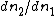varies within the spectrum. This fact was first ascertained by J. Fraunhofer, who defined the colors by means of the dark lines in the solar spectrum; and showed that the ratio of the dispersion of two glasses varied about 20% from the red to the violet (the variation for glass and water is about 50%). If, therefore, for two colors, a and b,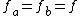, then for a third color, c, the focal length is different; that is, if c lies between a and b, then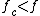, and vice versa; these algebraic results follow from the fact that towards the red the dispersion of the positive crown glass preponderates, towards the violet that of the negative flint. These chromatic errors of systems, which are achromatic for two colors, are called the secondary spectrum, and depend upon the aperture and focal length in the same manner as the primary chromatid errors do.

In fig. 11, taken from M. von Rohr's Theorie und Geschichte des photographischen Objectivs, the abscissae are focal lengths, and the ordinates wavelengths. The Fraunhofer lines used are shown in the table to the right of the figure.
 A' C D Green HgMercury (element)Mercury is a chemical element with the symbol Hg and atomic number 80. It is also known as quicksilver or hydrargyrum.... F G' Violet Hg. 767.7 656.3 589.3 546.1 486.2 454.1 405.1 nm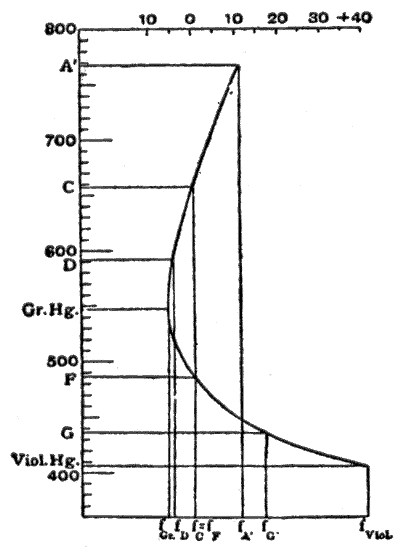The focal lengths are made equal for the lines C and F. In the neighborhood of 550 nm the tangent to the curve is parallel to the axis of wave-lengths; and the focal length varies least over a fairly large range of color, therefore in this neighborhood the color union is at its best. Moreover, this region of the spectrum is that which appears brightest to the human eye, and consequently this curve of the secondary on spectrum, obtained by making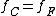, is, according to the experiments of Sir G. G. Stokes (Proc. Roy. Soc., 1878), the most suitable for visual instruments (optical achromatism,). In a similar manner, for systems used in photography, the vertex of the color curve must be placed in the position of the maximum sensibility of the plates; this is generally supposed to be at G'; and to accomplish this the F and violet mercury lines are united. This artifice is specially adopted in objectives for astronomical photography (pure actinic achromatism). For ordinary photography, however, there is this disadvantage: the image on the focusing-screen and the correct adjustment of the photographic sensitive plate are not in register; in astronomical photography this difference is constant, but in other kinds it depends on the distance of the objects. On this account the lines D and G' are united for ordinary photographic objectives; the optical as well as the actinic image is chromatically inferior, but both lie in the same place; and consequently the best correction lies in F (this is known as the actinic correction or freedom from chemical focus).

Should there be in two lenses in contact the same focal lengths for three colours a, b, and c, i.e.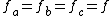, then the relative partial dispersion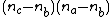must be equal for the two kinds of glass employed. This follows by considering equation (4) for the two pairs of colors ac and bc. Until recently no glasses were known with a proportional degree of absorption; but R. Blair (Trans. Edin. Soc., 1791, 3, p. 3), P. Barlow, and F. S. Archer overcame the difficulty by constructing fluid lenses between glass walls. Fraunhofer prepared glasses which reduced the secondary spectrum; but permanent success was only assured on the introduction of the Jena glasses by E. Abbe and O. Schott. In using glasses not having proportional dispersion, the deviation of a third colour can be eliminated by two lenses, if an interval be allowed between them; or by three lenses in contact, which may not all consist of the old glasses. In uniting three colors an achromatism of a higher order is derived; there is yet a residual tertiary spectrum, but it can always be neglected.

The Gaussian theory is only an approximation; monochromatic or spherical aberrations still occur, which will be different for different colors; and should they be compensated for one color, the image of another color would prove disturbing. The most important is the chromatic difference of aberration of the axis point, which is still present to disturb the image, after par-axial rays of different colors are united by an appropriate combination of glasses. If a collective system be corrected for the axis point for a definite wave-length, then, on account of the greater dispersion in the negative components — the flint glasses, — over-correction will arise for the shorter wavelengths (this being the error of the negative components), and under-correction for the longer wave-lengths (the error of crown glass lenses preponderating in the red). This error was treated by Jean le Rond d'Alembert, and, in special detail, by C. F. Gauss. It increases rapidly with the aperture, and is more important with medium apertures than the secondary spectrum of par-axial rays; consequently, spherical aberration must be eliminated for two colors, and if this be impossible, then it must be eliminated for those particular wave-lengths which are most effectual for the instrument in question (a graphical representation of this error is given in M. von Rohr, Theorie und Geschichte des photographischen Objectivs).

The condition for the reproduction of a surface element in the place of a sharply reproduced point — the constant of the sine relationship must also be fulfilled with large apertures for several colors. E. Abbe succeeded in computing microscope objectives free from error of the axis point and satisfying the sine condition for several colors, which therefore, according to his definition, were aplanatic for several colors; such systems he termed apochromatic. While, however, the magnification of the individual zones is the same, it is not the same for red as for blue; and there is a chromatic difference of magnification. This is produced in the same amount, but in the opposite sense, by the oculars, which Abbe used with these objectives (compensating oculars), so that it is eliminated in the image of the whole microscope. The best telescope objectives, and photographic objectives intended for three-color work, are also apochromatic, even if they do not possess quite the same quality of correction as microscope objectives do. The chromatic differences of other errors of reproduction have seldom practical importances.

### Britannica references

• H. D. Taylor, A System of Applied Optics (1906). The classical treatise in English.
• R. S. Heath, A Treatise on Geometrical Optics (2nd ed., 1895).
• L A. Herman, A Treatise on Geometrical Optics (1900).
• S. Czapski, Theorie der optischen Instrumente nach Abbe, published:
• separately at Breslau in 1893,
• as vol. ii of Winkelmann's Handbuch der Physik in 1894, and as
• S. Czapski and O. Eppenstein, Grundzuge der Theorie der optischen Instrumente nach Abbe (2nd ed., Leipzig, 1903).
• Moritz von Rohr
Moritz von Rohr
Moritz von Rohr was an optical scientist at Carl Zeiss in Jena.A street in Jena is named after him: Moritz-von-Rohr-Straße, near Carl-Zeiss-Promenade and Otto-Schott-Straße, reminders of the proud optical heritage of the city.-Life:...

, ed., Die bilderzeugung in optischen Instrumenten vom Standpunkte der geometrischen Optik (Berlin, 1904). The collection of the scientific staff of Carl Zeiss
Carl Zeiss
Carl Zeiss was a German maker of optical instruments commonly known for the company he founded, Carl Zeiss Jena . Zeiss made contributions to lens manufacturing that have aided the modern production of lenses...

at Jena
Jena
Jena is a university city in central Germany on the river Saale. It has a population of approx. 103,000 and is the second largest city in the federal state of Thuringia, after Erfurt.-History:Jena was first mentioned in an 1182 document...

, which contains articles by Arthur König
Arthur König
Arthur Peter König devoted his short life to physiological optics. Born with congenital kyphosis he studied in Bonn and Heidelberg, moving to Berlin in the fall of 1879 where he studied under Hermann von Helmholtz, whose assistant he became in 1882...

and M. von Rohr specially dealing with aberrations.